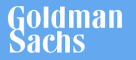New update is available. Click here to update.
Last Updated: 13 Nov, 2020

# Count Triplets

Easy+19 more companies

## Problem statement#### Your task is to return the number of triplets in the list that sum up to the value ‘X’.

##### Input format :
``````The first line contains a single integer ‘T’ denoting the number of test cases. The test cases follow.

The first line of each test case contains a single integer ‘X’.

The second line of the input contains the elements of the doubly linked list separated by a single space and terminated by -1. Hence, -1 would never be a list element.
``````
##### Output Format:
``````For each test case, print in a new line a single integer denoting the number of triplets that have the sum equal to ‘X’.
``````
##### Note:
``````You don’t need to print anything; it has already been taken care of. Just implement the given function.
``````
##### Constraints :
``````1 <= T <= 5
1 <= N <= 10 ^ 3
- 3 * 10 ^ 5 <= X <= 3 * 10 ^ 5
- 10 ^ 5 <= data <= 10 ^ 5 and data != - 1

Where ‘N’ is the number of nodes in the given linked list, and ‘X’ is the required triplet sum value.

Time limit: 1 sec
``````## Approaches

### 01 ApproachThe idea is to explore all the triplets and count those triplets which have a sum equal to ‘X’. We will use a variable 'COUNT' which will be initialized to 0, to count the number of triplets with sum ‘X’. The steps are as follows:

• We will iterate the linked list and pick each node one by one and pivot that node as the first element of the triplet, let this node be 'FIRSTPTR'.
• For the second element of the triplet, iterate from the next node of 'FIRSTPTR' till the end of the linked list and one by one pick each node and pivot that, let’s say this node is 'SECONDPTR'. So we will pivot 'SECONDPTR' as the second element of the triplet.
• For the third element, iterate from the next node of 'SECONDPTR' till the end of the list and pivot each node as the third element.
• If the sum of the three pivoted nodes is equal to ‘X’ increment 'COUNT' by 1.